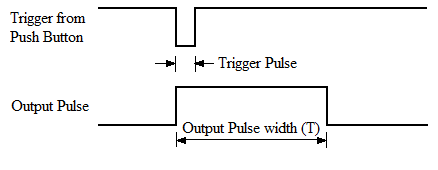# 555 Timer Monostable Calculator

This 555 timer monostable circuit calculator can be used to get the output pulse width (Delay time) for a 555 Timer monostable Circuit. In Monostable mode of 555 timer IC, when power is applied, the output remains low for the Delay time and then becomes high and remains high, or vice versa.

The time delay in a monostable mode is calculated as per the below formulae:
Output Pulse Width (secs) = 1.1 x R1 x C1

Enter any two known values and calculate the remaining one:

 Capacitor (C) microFarad (uF) nanoFarad (nf) Resistor (R) Ohm Kilo Ohm Mega Ohm Output Pulse Width (T) Seconds

### Concept of 555 Timer Monostable Circuit Calculator

555 Timer IC’s are the most commonly used ICs for timing and Pulse generation applications. They can adopt itself into various applications due to its different operating modes. The three main operating modes of a 555 Timer are Astable Mode, Monostable Mode and Bi-Stable Mode. Each mode has its own properties and applications since every mode provides a different type of wave forms.

During a Monostable mode, as the name suggest there will one (mono) stable high state of pulse for a pre-defined time. This pre-defined time can be set by selecting the correct values of Resistor (R1) and Capacitor (C1) shown in the below circuit.This is a simple circuit to make the 555 timer IC to work in Mont stable mode. Whenever the push button connected to Trigger pin (pin 2) is pressed a trigger pulse is detected by the 555 IC and so it will trigger the output pulse on its output pin (pin 3) as shown in the waveform below.This output pulse will stay high based on its pulse width. This output pulse width sets the pre-defined time and as said earlier it can be set by selecting the correct values of Resistor (R1) and Capacitor (C1) using the below formulae.

Output Pulse Width (secs) = 1.1 x R1 x C1

For the above circuit the value of R1 = 100k and value of C1 = 10uF, let us use the formulae to calculate the time in seconds

R1 = 100k = 100000 ohms

C1 = 10uF = 0.00001 Farads

So,  T = 1.1 * 100000 * 0.00001

T = 1.1 seconds

To avoid all this hassle of data conversion and calculation you can use the 555 timer monostable calculator given above to calculate the value of Time, or you can even calculate of R1 or C1 for any specific time duration. Just enter any two parameters leaving the third one blank and click on calculate to get you results.